2 4/6 + 1 3/6

Correct result:

f =  4.1667

#### Solution:

$f=\left(2+\frac{4}{6}\right)+\left(1+\frac{3}{6}\right)=\frac{25}{6}=4.1667$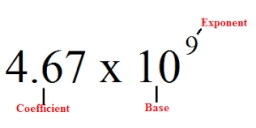We would be pleased if you find an error in the word problem, spelling mistakes, or inaccuracies and send it to us. Thank you!Tips to related online calculators
Need help calculate sum, simplify or multiply fractions? Try our fraction calculator.

## Next similar math problems:Why does 1 3/4 + 2 9/10 equal 4.65? How do you solve this?3 3/4 + 2 3/5 + 5 1/2 Show your solution.Add this two mixed numbers: 1 5/6 + 2 2/11=Add marks (+, -, *, /, brackets) to fullfill equations 1 3 6 5 = 10 This is for the 4th grade of the primary school - with no negative numbers yet
• Simplify 3Simplify mixed numerals expression: 8 1/4- 3 2/5 - (2 1/3 - 1/4) Show your solution.
• Mixed numbersRewrite mixed numbers so the fractions have the same denominator: 5 1/5 - 2 2/32 and 1 8th plus 1 and 1 3rd =
• Expression plus minusEvaluate expression: (-1)2 . 12 – 6 : 3 + (-3) . (-2) + 22 – (-3) . 2
• Expression 8Evaluate this expressions: a) 5[3 + 4(2.8 - 3)] b) 5×(8-4)÷4-2
• The perimeter 2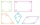The perimeter of the quadrilateral a = 1m b = 14/5m c = 2 3/10m d = 1 4/5m?
• Expression 1What is 7+8-(5×2)+5-4+(6×(5-3)+6)-(8+10)-7+6?
• How many 3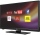How many hours the Andersons watched TV in all Wednesday 3/1 hr Thursdays 2/3 hr Friday 4/5 hr Saturday 3/4 hr
• FractionsSort fractions ? by its size. Result write as three serial numbers 1,2,3.
• On the floor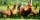You enter the room and there are 2 dogs, 4 horses, 1 giraffe and one duck on the bed, 3 chickens are flying over the chair. How many feet are on the floor?What is 4 1/2+2/7-213/14?3 pounds subtract 1/3 of a pound.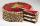Thomas ate 1/3 of cake, Bohouš of the rest of the cake ate 2/5. What fraction of cake left over for others?